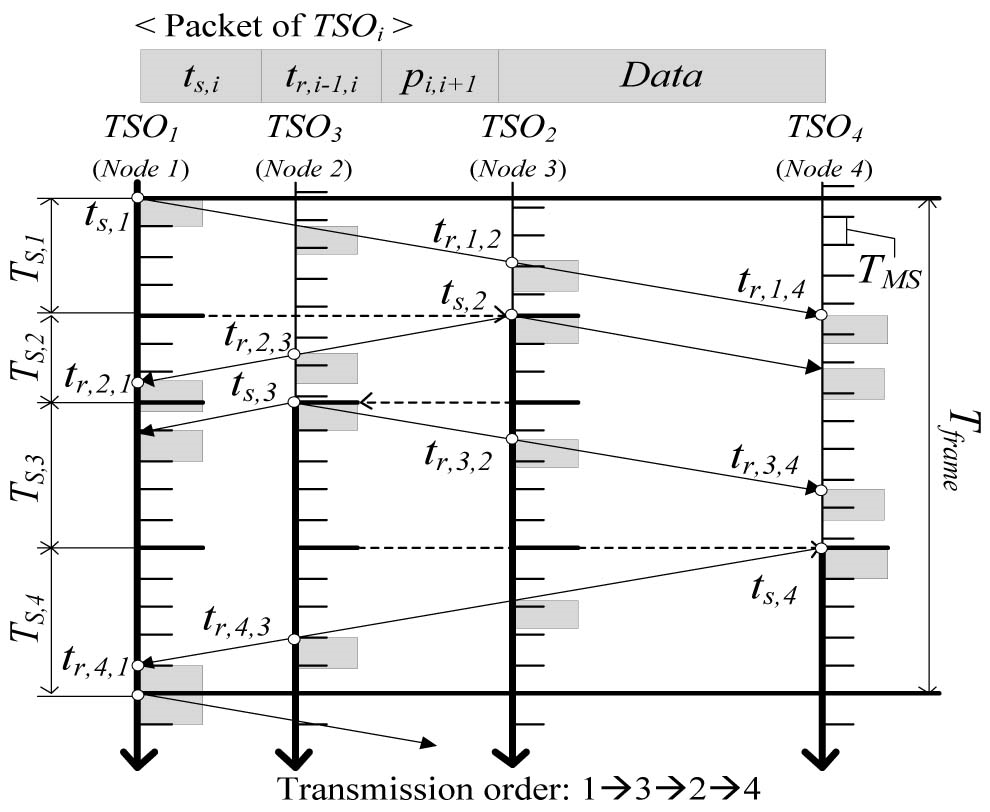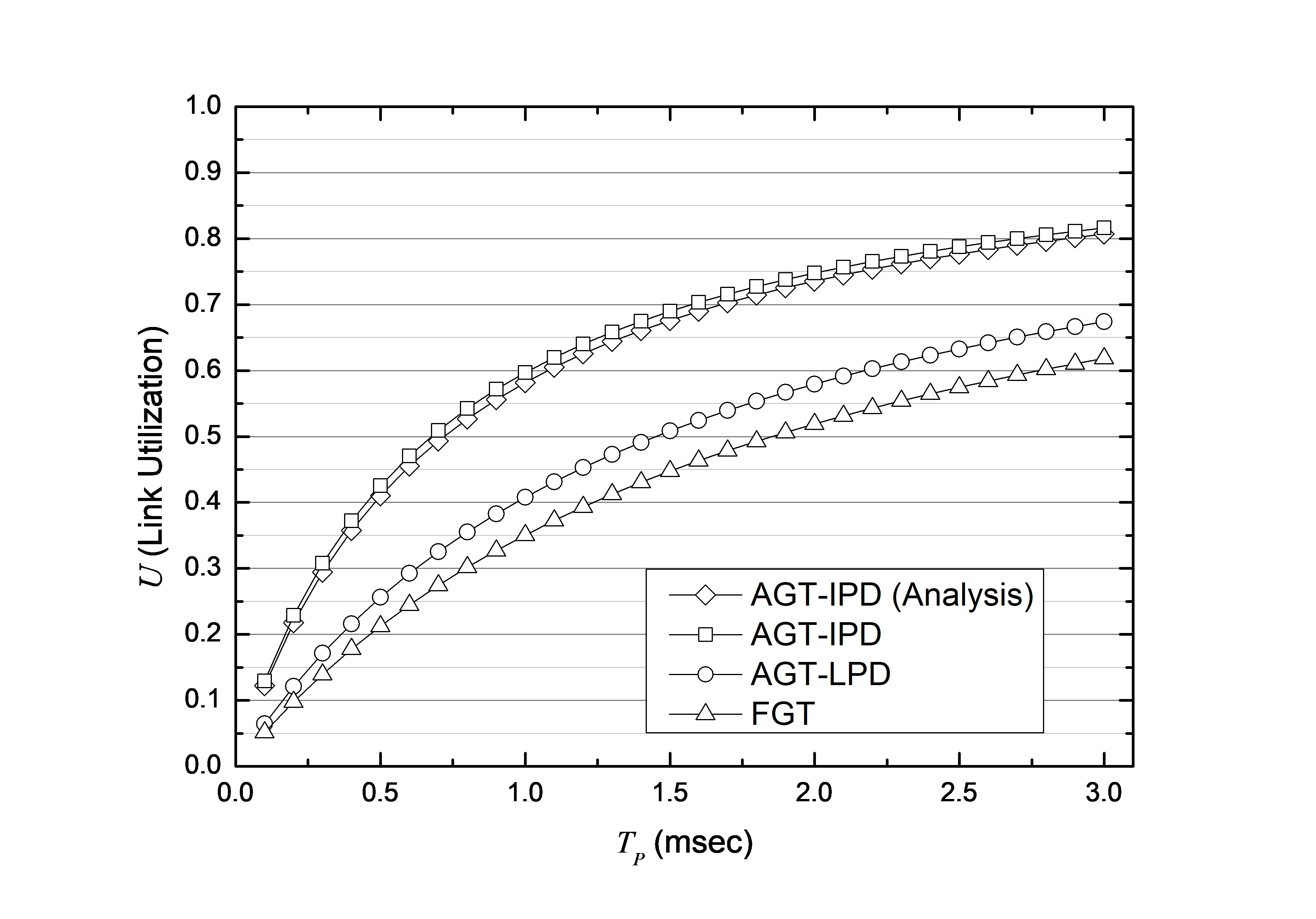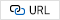Mobile QR Code1. (School of Computer Science and Engineering, Kyungpook National University / Daegu 41566, Korea {duddmd0219, neloyou}@knu.ac.kr )

## 1. Introduction

Most military networks need to broadcast shared data to every friendly force. Thus, TDMA is widely used in military networks because it adequately ensures broadcasting without collisions by allocating the same frequency to each user in a time slot. In particular, airborne military networks require guard time to prevent collisions owing to the large propagation delay. However, use of a guard time causes waste and degrades network performance, but reducing the time interval while ensuring broadcasting is a challenge.

In this paper, we propose Adaptive Guard Time with Inter-transmitter Propagation Delay (AGT-IPD), a scheme for reducing guard time while ensuring broadcasting. AGT-IPD does not require a central node, and assumes that all nodes are synchronized and share prior information (e.g., propagation delay). Each transmitter adjusts the guard time considering the propagation delay for the next time slot owner, referred to as PD-NEXT. AGT-IPD also provides a distributed mechanism that uses PD-NEXT for propagation delay estimation and time synchronization. AGT-IPD does not provide tight time synchronization. Thus, we consider additional guard time to compensate for errors due to rough time synchronization. We consider a network initialization mode in which all nodes are unsynchronized and use a fixed guard time. After network initialization mode, each node adjusts the guard time adaptively by using PD-NEXT, which was estimated in the previous frame. We constructed analytical and simulated models of AGT-IPD, showing through numerical results that AGT-IPD provides higher link utilization than other schemes, regardless of packet length, maximum communication range, the number of nodes, and the number of errors.

## 2. Related Works

Most studies have focused solely on reducing the guard time in underwater wireless sensor networks (UWSNs) [1-4]. UWNSs are shaped as tree-based multi-hop networks. The objective of a UWSN is to deliver data to the sink node for ocean exploration, monitoring tasks, and target tracking. To achieve high link utilization when changing data between nodes, the guard time is set longer than the transition time in the modem . By setting the optimal time slot length, the guard time is reduced to reach efficient throughput. However, the scheme does not ensure broadcasting, and a way to reduce guard interval itself was not devised. The scheme proposed in  considers how to select the nearest node and how to broadcast between adjacent nodes, but assumes that each sensor is fixed. In these schemes, the TDMA method provided is not suitable for military networks that must handle dynamic movement and broadcast tactical data.

In general, military networks require high reliability and stability. The traditional centralized TDMA, a method in which a particular node allocates a time slot centrally, is likely to paralyze the entire network if a problem arises with the node managing the resource. On the other hand, distributed TDMA is a method of allocating distributed time slots without a separate node. Therefore, research on tactical data links has focused on distributed TDMA techniques rather than centralized TDMA. Distributed TDMA has been studied in various fields. The scheme in  proposes efficient time slot adjustment to minimize vehicle-to-vehicle collisions in a vehicle ad hoc network. The authors in  showed that localization accuracy is achieved by quickly allocating all idle time slots to maximize channel ranging and communication in mobile UWB networks.

There are several types of tactical data link, among which Link-16 is a representative airborne military communication system with a maximum range of 300 nautical miles (nm) . It uses a fixed guard time considering the maximum propagation delay in accord with the maximum communication range. In the worst-case scenario, it causes an unnecessary waste of time when communicating between nodes at short distances, and leads to poor network performance. In , an optimal TDMA scheme that uses an adaptive guard time considering the propagation delay from the time slot owner to its farthest neighbor was defined. However, this scheme does not provide optimal performance, and assumes that each node has already determined the propagation delay to its farthest neighbor. To avoid ambiguity, we refer to that scheme as AGT-LPD.

## 3. The Proposed Scheme

We consider a single-hop, distributed TDMA network with a large propagation delay. The network consists of $\textit{N}$ nodes that are unsynchronized. We do not consider a time slot allocation procedure. We focus solely on guard time control and time synchronization by using PD-NEXT. Therefore, we assume that each node has one time slot per frame according to a predefined order, and that order is already stored in all nodes before the system starts, as in Link-16 . We also assume that all nodes transmit packets of the same length.

We define a number of variables. A frame consists of $\textit{N}$ time slots, each of which consists of several mini-slots. Let $\textit{T}$$_{frame} denote the frame length. We can express \textit{T}$$_{frame}$ as

##### (1)
$T_{\textit{frame}}=\sum _{i=1}^{N}T_{S,i}$

##### Fig. 1. Example of the AGT-IPD frame and packet structure in normal mode.In each frame, guard time control and time synchronization are performed with PD-NEXT, which was estimated in the previous frame. However, in the first frame, none of the nodes can perform these procedures because PD-NEXT has not yet been estimated. Therefore, AGT-IPD provides two modes: network initialization mode and normal mode.

### 3.1 Network Initialization Mode

The network initialization mode is used for estimating PD-NEXT while all nodes are unsynchronized in the first frame. $\textit{TSO}$$_{1} starts the network by broadcasting a packet with \textit{t}$$_{s,1}$. As soon as each node receives the packet, it sets the local time to $\textit{t}$$_{s,1} and starts the first frame. Because all nodes are still unsynchronized, a fixed guard time is used to prevent collisions, and its length is denoted as \textit{T}$$_{MAX\backslash \_ RTT}$, which is the maximum round trip time.

After the first time slot, each node estimates PD-NEXT by exchanging a packet. The packet of $\textit{TSO}$$_{i} contains \textit{t}$$_{s,i}$ and $\textit{t}$$_{r,i-1,i} as well as the data. In the \textit{i-th} time slot, \textit{TSO}$$_{i}$ broadcasts the packet, and $\textit{TSO}$$_{i+1} records \textit{t}$$_{r,i,i+1}$. Subsequently, in the ($\textit{i+1}$)$\textit{-th}$ time slot, $\textit{TSO}$$_{i+1} broadcasts the packet containing \textit{t}$$_{s,i+1}$ and $\textit{t}$$_{r,i,i+1} as well as the data. Then, \textit{TSO}$$_{i}$ records $\textit{t}$$_{r,i+1,i} and obtains \textit{p}$$_{i,i+1}$ with the following calculation: {($\textit{t}$$_{r,i+1,i} - \textit{t}$$_{s,i}$) - ($\textit{t}$$_{s,i+1} - \textit{t}$$_{r,i,i+1}$)}/2. However, $\textit{TSO}$$_{i+1} does not yet have \textit{p}$$_{i,i+1}$. In particular, $\textit{TSO}$$_{N} regards \textit{TSO}$$_{1}$ as the next time slot owner in order to estimate PD-NEXT.

### 3.2 Normal Mode

After network initialization mode (i.e., for the first frame), all nodes switch to normal mode. All nodes perform guard time control and time synchronization with PD-NEXT estimated in the previous frame. In addition, all nodes estimate PD-NEXT to be used in the next frame.

A packet of $\textit{TSO}$$_{i} contains \textit{t}$$_{s,i}$, $\textit{t}$$_{r,i-1,i}, and \textit{p}$$_{i,i+1}$ as well as the data, as shown in Fig. 1. When $\textit{TSO}$$_{i+1} receives this packet, it obtains \textit{t}$$_{s,i}$ and $\textit{p}$$_{i,i+1}. Thus, \textit{TSO}$$_{i+1}$ can achieve time synchronization with $\textit{TSO}$$_{i} by setting the local time as the sum of \textit{t}$$_{s,i}$ and $\textit{p}$$_{i,i+1}. It also determines \textit{t}$$_{s,i+1}$ as follows:

##### (3)
$t_{s,i+1}=t_{s,i}+T_{S,i}$

## 4. Models for Simulation and Analysis

In this section, we describe the simulation model and the analytical model for AGT-IPD. We use link utilization as the performance metric. Let $\textit{U}$ denote link utilization during one frame in normal mode. We obtain $\textit{U}$ with

##### (5)
$U=\frac{N\times T_{P}}{T_{\textit{frame}}}$

##### Fig. 2. Comparison of link utilizations according to TP (R = 300 nm, N = 216, $e_{MAX}$ = 0).Fig. 3 shows $\textit{U}$ according to $\textit{R}$ when $\textit{N}$ = 216 and $\textit{e}$$_{MAX} = 0 ms. In each time slot, the ratio of \textit{T}$$_{P}$ to guard time length, which is affected by $\textit{R}$, affected $\textit{U}$ directly. When $\textit{R}$ was 300 nm, the maximum propagation delay was 1.852 ms. We considered two types of packet: one with a length shorter than the maximum propagation delay (0.1 ms) and another with a length that is longer (3.0 ms). As shown in Fig. 3, $\textit{U}$ was high when $\textit{T}$$_{P} was large. Regardless of \textit{T}$$_{P}$, AGT-IPD consistently provided the highest $\textit{U.}$ The analytical and simulated models of AGT-IPD also showed almost identical performance.

Fig. 4 shows $\textit{U}$ according to $\textit{N}$$_{D} when \textit{R} = 300 nm and \textit{e}$$_{MAX}$ = 0 ms. When $\textit{N}$$_{D} was less than 5, AGT-LPD was only affected by \textit{N}$$_{D}$. Regardless of $\textit{T}$$_{P}, AGT-IPD and FGT were hardly affected by \textit{N}$$_{D}$. AGT-IPD also provided the highest $\textit{U}$.

### ACKNOWLEDGMENTS

This research was supported by Kyungpook National University Research Fund, 2021.

### REFERENCES

1
Anjangi P., Chitre M., 2015, Design and Implementation of Super-TDMA: A MAC Protocol Exploiting Large Propagation Delays for Underwater Acoustic Networks, In Proceedings of the 10th International Conference on Underwater Networks & Systems (WUWNET '15), Article 1, pp. 1-82
Hsu C-C., Lai K-F., Chou C-F., Lin K. C-J., 2009, ST-MAC: Spatial-Temporal MAC Scheduling for Underwater Sensor Networks, IEEE INFOCOM, pp. 1827-18353
Cerqueira L.S., Vieira A.B., Vieira L.F.M., et al. , 2021, A cooperative protocol for pervasive underwater acoustic networks, Wireless Netw 27, pp. 1941-19634
Kredo II K., Djukic P., Mohapatra P., 2009, STUMP: Exploiting Position Diversity in the Staggered TDMA Underwater MAC Protocol, IEEE INFOCOM, pp. 2961-29655
Johari S., Krishna M., 2021, TDMA based contention-free MAC protocols for vehicular ad hoc networks: A survey, Vehicular Communications, Vol. 28, pp. 1003086
Cao Y., Chen C., St-Onge D., Beltrame G., 1 Sept.1, 2021, Distributed TDMA for Mobile UWB Network Localization, in IEEE Internet of Things Journal, Vol. 8, No. 17, pp. 13449-134647
Baek H., Lim J., 2016, Performance analysis of Block ACK-Based Slotted ALOHA for wireless networks with long propagation delay, Ad Hoc Networks, Vol. 42, pp. 34-468
Spherical coordinate system, (accessed July. 10, 2022). Website Link9
Highnam P. T., 2002, Tactical Targeting Network Technologies (TTNT), DARPATech2002 Symposium## Author

Youngeun Cho is currently pursuing a B.S. from the School of Computer Science and Engineering at Kyungpook National University in Daegu, South Korea, and graduates in February 2023. Her research interests include wireless communications and networks, UAV systems, and military communications and networks.

Hoki Baek received his B.S, M.S., and Ph.D. from the Department of Computer Science at Ajou University in Suwon, South Korea, in 2006, 2008, and 2014, respectively. From March 2014 to February 2015, he served as a full-time researcher at Ajou University's Jangwee Defense Research Institute, and from March 2015 to February 2021, he was a Lecture Professor in the Department of Military and Digital Convergence at Ajou University. Currently, he is an Assistant Professor for the School of Computer Science and Engineering at Kyungpook National University. He is a life member and a director of the Korean Institute of Communications and Information Sciences (KICS), and is an editorial board member for the Journal of the Korean Institute of Communications and Information Sciences (J-KICS). He is a member of the Defense Information Technology Standards (DITA) Standard Working Group (SWG) for the Ministry of National Defense, serving from June 2020 to May 2024. His research interests include 5G/6G communications and networks, UAV networks, Wi-Fi, IoT, military communications and networks, and positioning and time synchronization.